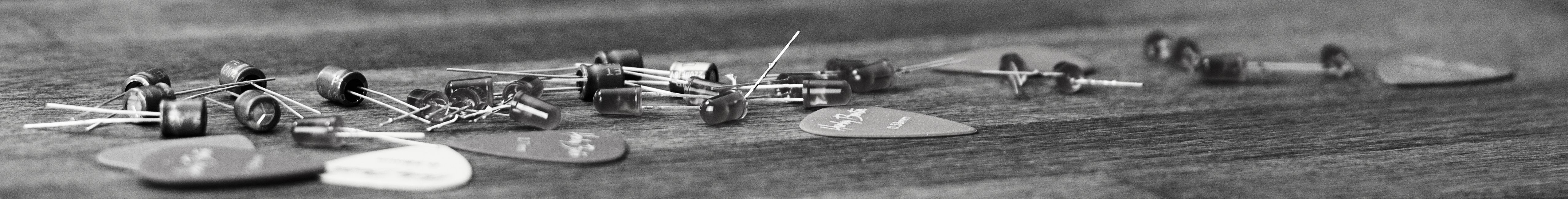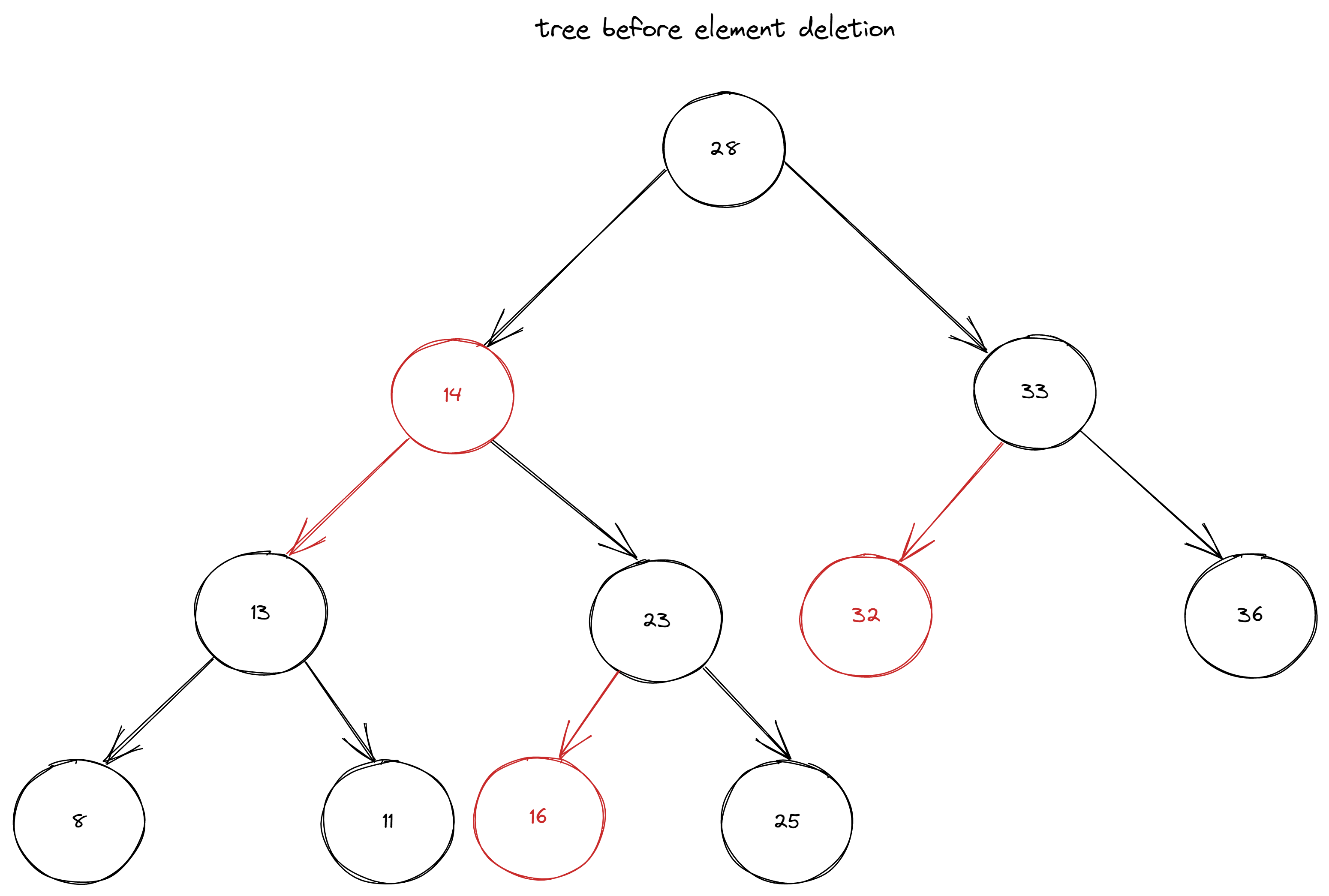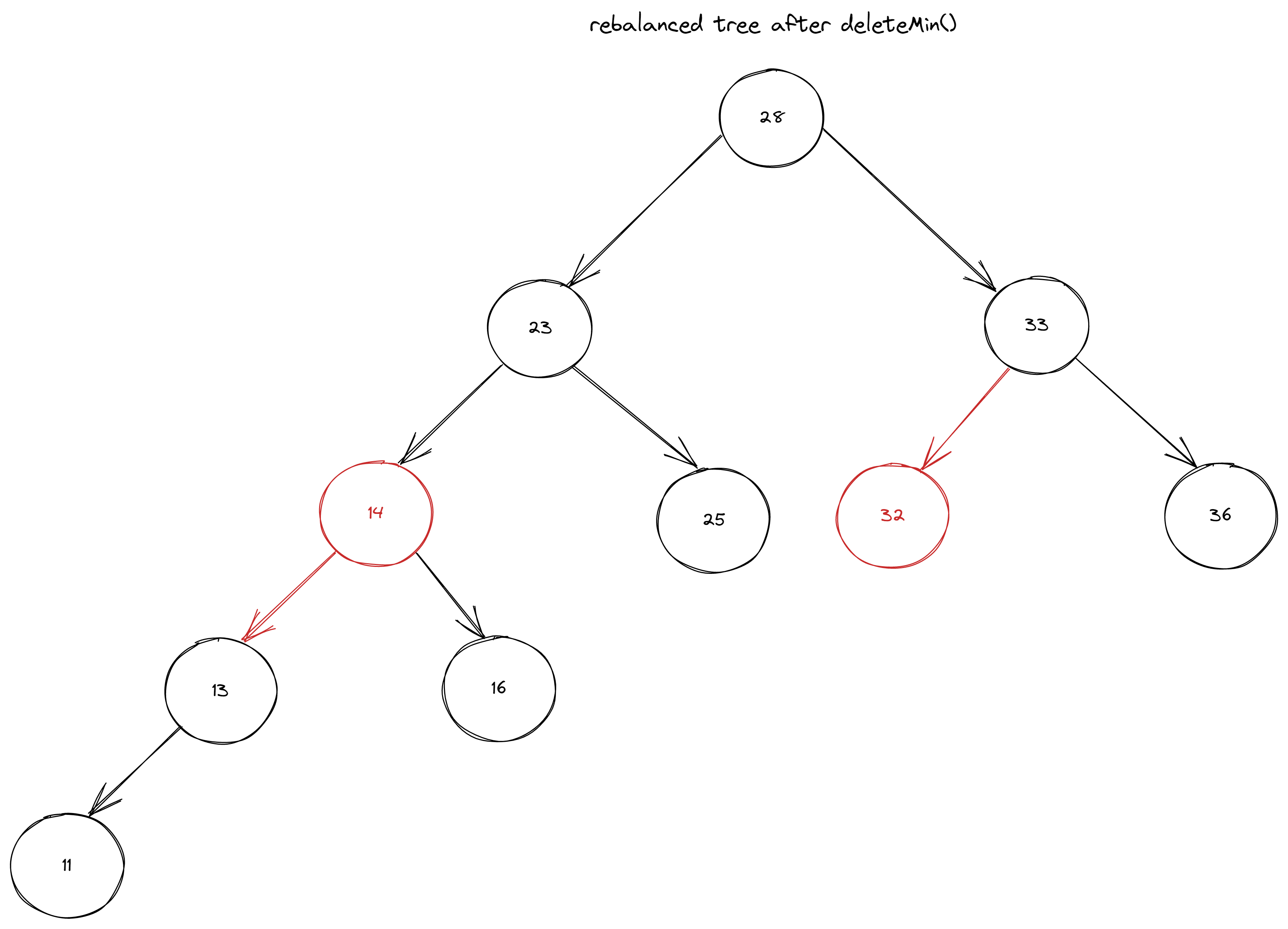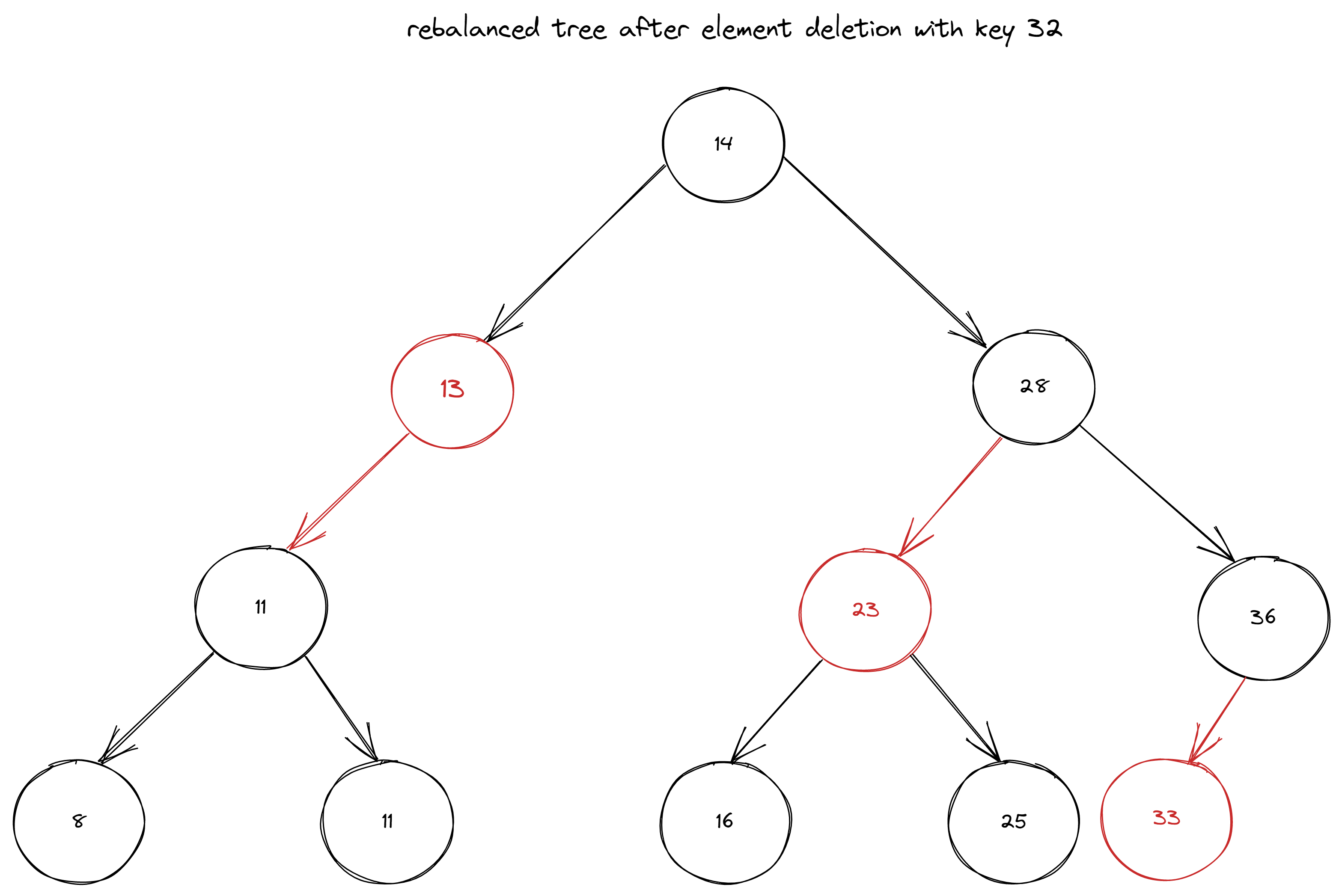savchenko.tech / software engineering blog by Maryna Savchenko## Red Black Binary Search Tree: Part 2 delete. Algorithms series

2022-07-28

To implement delete() method we need to take care about helper methods first. First we will take a look at deleteMin().

### DELETE MIN

Test cases for deleteMin() should check that:

• minimal element is not present
• tree is balanced, which means:
• there is no more than 1 left RED links in the row
• there is no right RED link
• root should be always BLACK
``````    @Test
void shouldDeleteMinElement() {
RedBlackBinarySearchTree<Integer, String> brBinarySearchTree = createRedBlackBST();

brBinarySearchTree.deleteMin();

assertNull(brBinarySearchTree.get(8));
}

@Test
void treeShouldBeRebalancedAfterDeleteMin() {
RedBlackBinarySearchTree<Integer, String> brBinarySearchTree = createRedBlackBST();

brBinarySearchTree.deleteMin();

assertEquals("23", brBinarySearchTree.root.left.value);
assertFalse(brBinarySearchTree.root.left.color, "Should be black");

assertEquals("14", brBinarySearchTree.root.left.left.value);
assertTrue(brBinarySearchTree.root.left.left.color, "Should be red");

assertEquals("13", brBinarySearchTree.root.left.left.left.value);
assertFalse(brBinarySearchTree.root.left.left.left.color, "Should be black ");

assertEquals("25", brBinarySearchTree.root.left.right.value);
assertFalse(brBinarySearchTree.root.left.right.color, "Should be black");
}
``````2 more helper methods for achieving balance will be moveRedLeft() and moveRedRight().

``````private Node moveRedLeft(Node node) {
flipColors(node);
if (isRed(node.right.left)) {
node.right = rotateRight(node.right);
node = rotateLeft(node);
flipColors(node);
}
return node;
}
``````
``````    private Node moveRedRight(Node node) {
flipColors(node);
if (isRed(node.left.left)) {
node = rotateRight(node);
flipColors(node);
}
return node;
}
``````

With helper methods in place we can move on with implementation.

``````    public void deleteMin() {
if (!isRed(root.left) && !isRed(root.right))
root.color = RED;

root = deleteMin(root);
if (root != null) root.color = BLACK;
}

private Node deleteMin(Node node) {
if (node.left == null)
return null;

if (!isRed(node.left) && !isRed(node.left.left))
node = moveRedLeft(node);

node.left = deleteMin(node.left);
return balanceBST(node);
}
``````### DELETE

Test cases for delete() will be similar to deleteMin(). They should check:

• given key is not present
• tree is rebalanced
``````    @Test
void shouldDeleteElementByKey() {
RedBlackBinarySearchTree<Integer, String> brBinarySearchTree = createRedBlackBST();

brBinarySearchTree.delete(32);

assertNull(brBinarySearchTree.get(32));
}

@Test
void treeShouldBeRebalancedAfterDelete() {
RedBlackBinarySearchTree<Integer, String> brBinarySearchTree = createRedBlackBST();

brBinarySearchTree.delete(32);
assertEquals("14", brBinarySearchTree.root.value);

assertEquals("28", brBinarySearchTree.root.right.value);
assertFalse(brBinarySearchTree.root.right.color, "Should be black");

assertEquals("23", brBinarySearchTree.root.right.left.value);
assertTrue(brBinarySearchTree.root.right.left.color, "Should be red");

assertEquals("33", brBinarySearchTree.root.right.right.left.value);
assertTrue(brBinarySearchTree.root.right.right.left.color, "Should be red");
}
``````

Deletion implementation is based on deletion of simple BST. It gets a bit more complicated because we need to keep the balance.

``````    public void delete(Key key) {
if (!isRed(root.left) && !isRed(root.right))
root.color = RED;

root = delete(root, key);
if (root != null) root.color = BLACK;
}

private Node delete(Node node, Key key) {
if (key.compareTo(node.key) < 0) {
if (!isRed(node.left) && !isRed(node.left.left))
node = moveRedLeft(node);
node.left = delete(node.left, key);
} else {
if (isRed(node.left))
node = rotateRight(node);
if (key.compareTo(node.key) == 0 && (node.right == null))
return null;
if (!isRed(node.right) && !isRed(node.right.left))
node = moveRedRight(node);
if (key.compareTo(node.key) == 0) {
Node x = min(node.right);
node.key = x.key;
node.value = x.value;
node.right = deleteMin(node.right);
} else node.right = delete(node.right, key);
}
return balanceBST(node);
}
``````Complete implementation with test cases can be found on GitHub.

Sources: